# 一、前言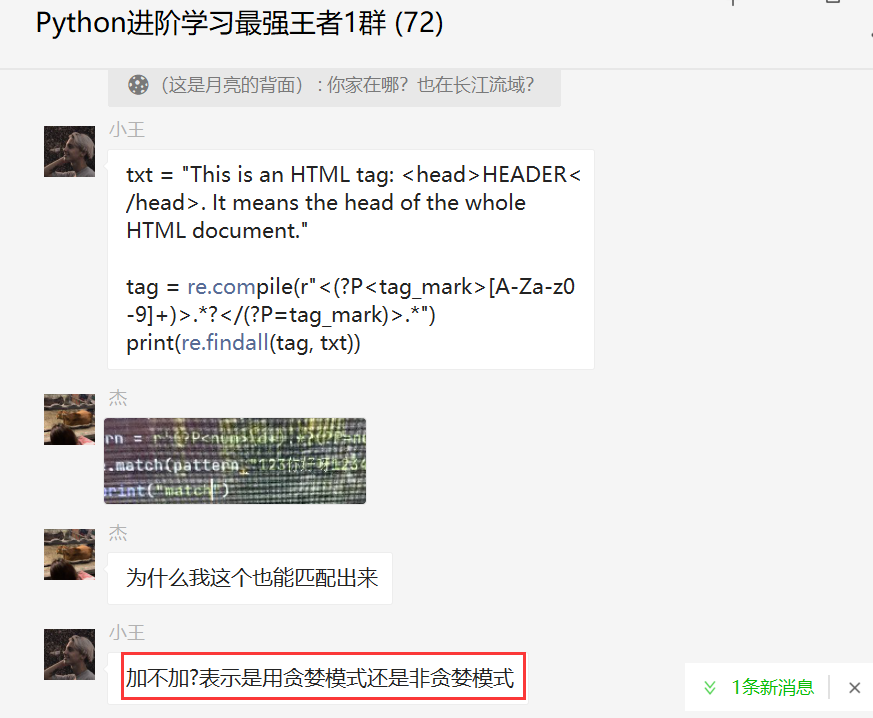# 二、解决过程

``````import re

pattern1 = re.compile(r"<.*>")
pattern2 = re.compile(r"<.*?>")
result1 = re.findall(pattern1, txt)
result2 = re.findall(pattern2, txt)
print(result1)
print(result2)
``````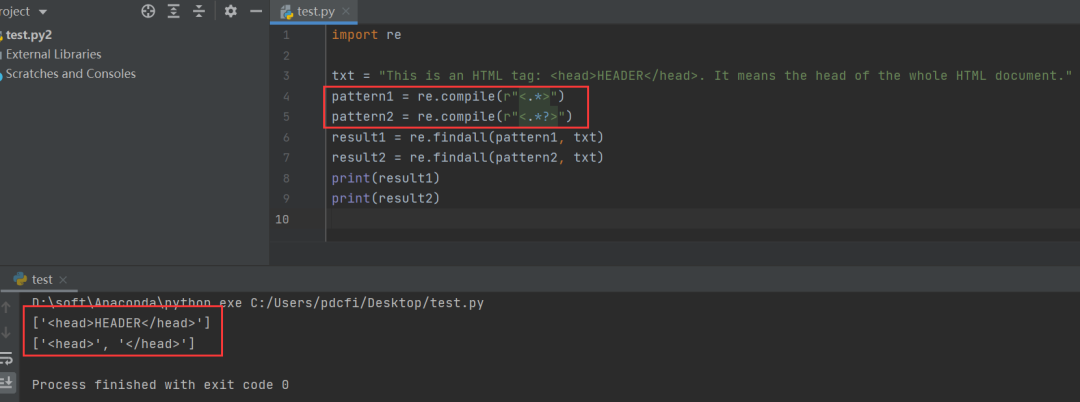``````pattern1 = re.compile(r"<.*>")
pattern2 = re.compile(r"<.*?>")
``````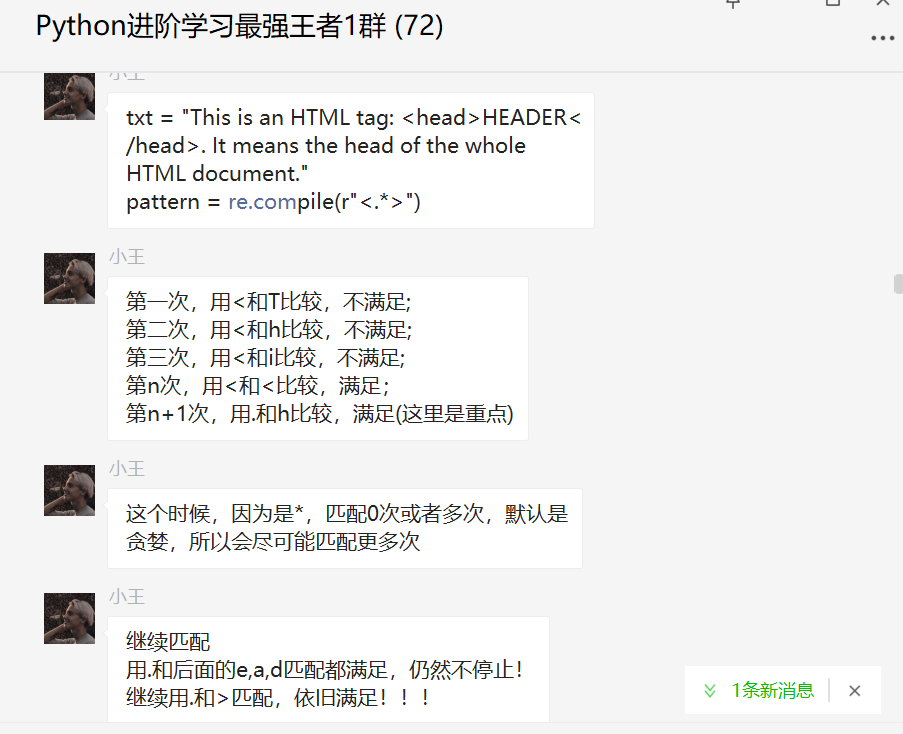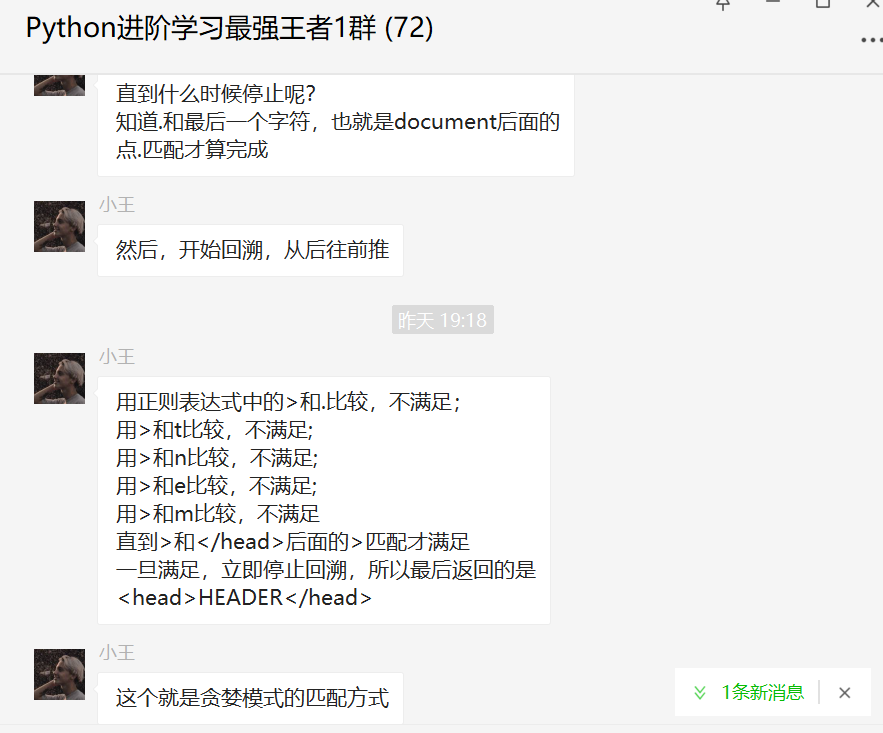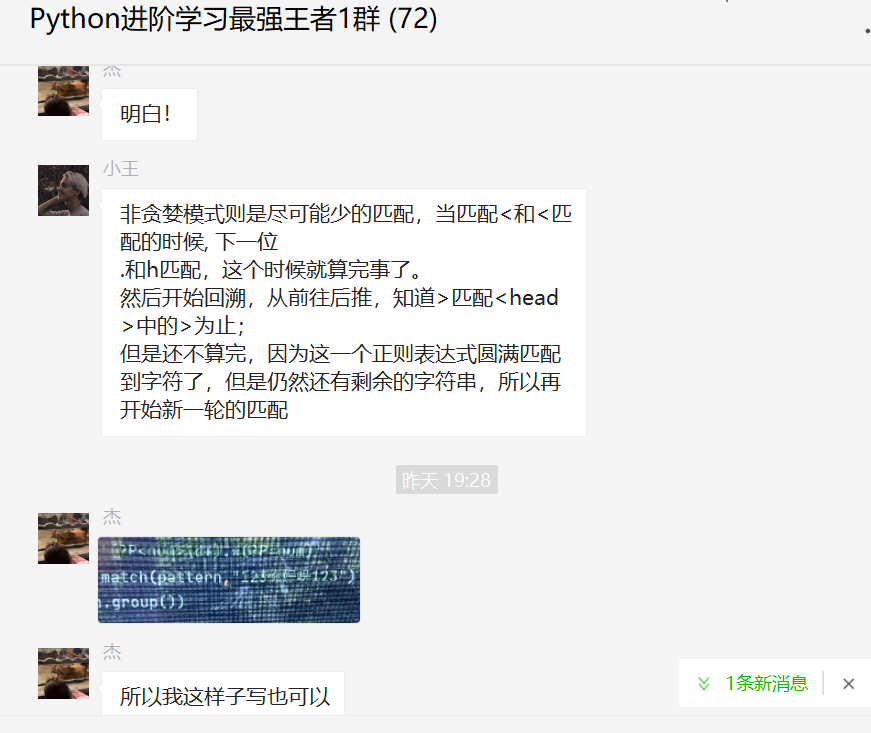# 小彩蛋

``````import re

tag = re.compile(r"<([A-Za-z0-9]+)>.*?</\1>.*")
print(re.findall(tag, txt))
``````

``````txt = "This is an HTML tag: <head>HEADER</head>. It means the head of the whole HTML document."

tag = re.compile(r"<(?P<tag_mark>[A-Za-z0-9]+)>.*?</(?P=tag_mark)>.*")
print(re.findall(tag, txt))
``````